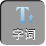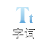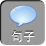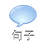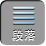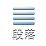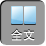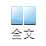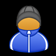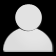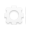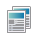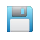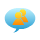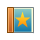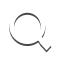-AA+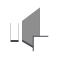1）定义：当两个复数实部相等，虚部互为相反数时，这样的两个复数叫做互为共轭复数.复数 z的共轭复数用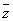来表示，即当 z= a+ bi时，= a-bi.

2）与模有关的性质：①| z|=||；② z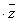= a 2+ b 2=| z| 2=|| 2.

①由模的定义可知，| z|=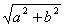，||=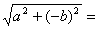，所以有| z|=||；②由复数的运算性质可知： z= a 2+ b 2=| z| 2=|| 2.

3）运算性质：①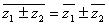；②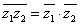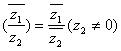；③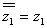.

①设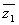= a+ bi,= c+ diabcdR）则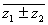=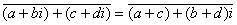=（ a+ c-b+ di=（ a-bi）+（ c-di）=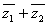，即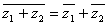，同理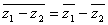.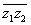=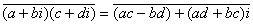=（ ac-bd-ad+ bci，而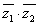=（ a-bi）（ c-di）=（ ac-bd-ad+ bci，故，同理.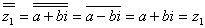4）重要结论：① zR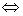z=；②非零复数 z是纯虚数z+= 0.